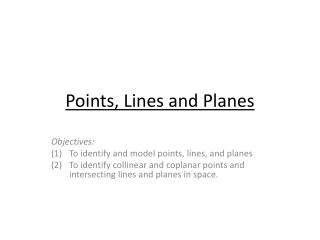DownloadDownload PresentationPoints , Lines and Planes

# Points , Lines and Planes

Download Presentation## Points , Lines and Planes

- - - - - - - - - - - - - - - - - - - - - - - - - - - E N D - - - - - - - - - - - - - - - - - - - - - - - - - - -
##### Presentation Transcript

1. Points, Lines and Planes Objectives: To identify and model points, lines, and planes To identify collinear and coplanar points and intersecting lines and planes in space.

2. Definitions • Point: a basic undefined term of geometry. A point is a location. In a figure, points are represented by a dot. Points are named by capital letters. • Line: A basic undefined term of geometry. A line is made up of points and has no thickness or width. In a figure, a line is shown with an arrowhead at each end. Lines are usually named by lowercase script letters or by writing capital letters for two points on a line, with a double arrow over the pair of letters. • Plane: A basic undefined term of geometry. A plane is a flat surface made up of points that has no depth and extends indefinitely in all directions. In a figure, a plane is often represented by a shaded, slanted 4-sided figure. Planes are usually named by a capital script letter or by three non-collinear points on the plane.

3. Definitions (continued) • Collinear: Points that are on the same line. • Coplanar: Points that are on the same plane • Undefined term: Words, usually readily understood, that are not formally explained by means of more basic words and concepts. The basic undefined terms in geometry are point, line, and plane • Space: A boundless 3-dimensional set of all points.

4. Point • “A location” • Represented by a dot • Named with a capital letter! • Can be referred as: A or point A • Has no thickness A •

5. Line • A string of points extending infinitely in two directions • Has arrowheads on both ends. • Named with a lower case letter… • Named b or line b • …Or with any TWO points on the line with a double headed arrow above it. • Named AB or BA b A B

6. Name the Lines • • Give 6 Possible names for the line: • AB, BA, BC, CB, AC, CA • List all possible names for the line: • WX, XW, WY, YW, WZ, ZW, XY, YX, XZ, ZY, YZ, ZY • Name the line: • d C B A W Y • • • • X Z d

7. Understanding Points and Lines B C A • A, B, and C are said to be: • Collinear • Are A, B, and D collinear? • No • • • • D

8. Planes A D • • A “flat surface” • Represented by a parallelogram • Name by a capital letter; or 3 non-collinear points. • Plane A or Plane BCD • Has no thickness • C • B

9. Understanding Planes A D • • Name the plane with 1 letter: • Plane A • Name the plane by its points: • Plane BCE • Are B, C, E collinear? • No • Are B,C,D, and E coplanar? • Yes • Are B, C, D, and F coplanar? • No • C • B • E • F

10. Interpret Sketches D • A • How many planes appear in this figure? - 4; Plane FDG, Plane FED, Plane FEG, Plane T Name 3 collinear points: - A, B, C Are points B, E, G, and D coplanar? Explain: - No, D is not on plane T whereas B, E and G are on plane T. Where does AC intersect Plane T? - At B. E • B • • G • F T C •